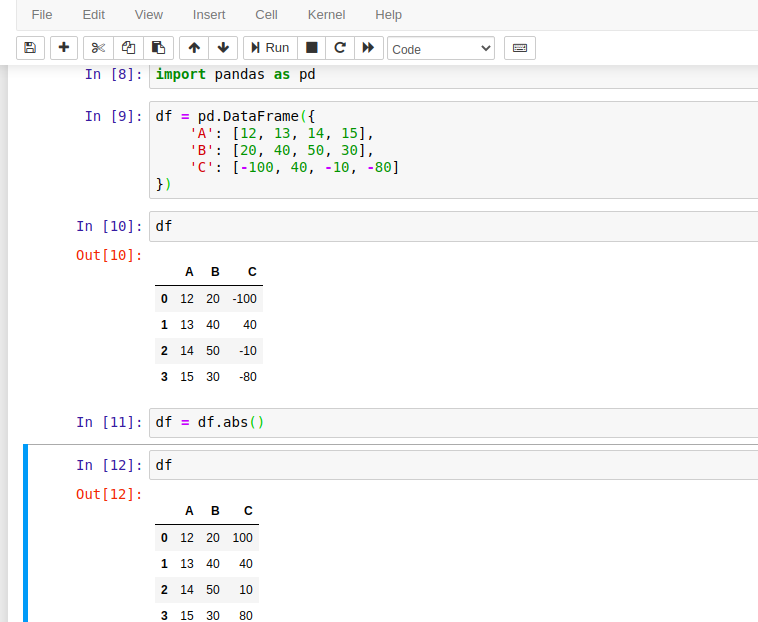# Pandas DataFrame abs() Method

Pandas abs() method is a built-in method of DataFrame that is used to get a DataFrame with the absolute numeric values. Absolute value is a non-negative value which is also known as the modulus in mathematics.

We can use this abs() method with numerical values only. So the DataFrame must contain only numerical values.

## Syntax

``DataFrame.abs()``

### Parameters:

It does not take any parameter.

### Return Value:

It returns a DataFrame containing the absolute value of each element.

## Example with Jupyter Notebook

Let's take an example to understand the use of pandas.DataFrame.abs() method. Following is the code that executed by using Jupyter notebook. If you are familiar with Jupyter, See the screenshot below.## Example: Get DataFrame of Absolute Values

A DataFrame that contains negative values can be turned into non-negative values by using the DataFrame.abs() method. See the example here. This example is similar to the above code, executed by using Jupyter notebook. If you are not familiar with Jupyter then use this code to understand.

``````# import pandas library
import pandas as pd
# Create DataFrame of data
df = pd.DataFrame({
'A': [12, 13, 14, 15],
'B': [20, 40, 50, 30],
'C': [-100, 40, -10, -80]
})
# print dataframem
print(df)
# get absolute value
df = df.abs()
print("New Dataframe of absolute values...")
# print new dataframe
print(df)
``````

Output:

A   B    C
0  12  20 -100
1  13  40   40
2  14  50  -10
3  15  30  -80

New Dataframe of absolute values...

A   B    C
0  12  20  100
1  13  40   40
2  14  50   10
3  15  30   80

Trending